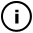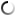# Monday, May 31, 2010

## honey

// use the mouse

// was thinking of a hexagonal memory
// directions, but got sidetracked
// after a glass of hungarian wine

void draw_cell(int x,int y,float size)
{
int sides=6;
beginShape();
for (int i=1; i<=sides; i++)
{
float a=(i/(float)sides)*2*PI;
vertex(x+size*sin(a),
y+size*cos(a));
}
endShape();
}

void draw_honeycomb(int w, int h, int f)
{
for (int x=0; x<w; x++)
{
for (int y=0; y<h; y++)
{
int offset=0;
if (y%2==0) offset=28;
draw_cell(50+x*57+offset,
50+y*50,
sin(x*mouseX*0.002+
f*0.05)*30);
}
}
}

void setup()
{
background(255);
size(500,300);
}

int t=0;
void draw()
{
fill(255);
stroke(255);
rect(0,0,800,300);
fill(0);
draw_honeycomb(7,5,t);
t++;
}

## infosubmitted by: slubbery
views: 300

Tags: honeycomb, red_wine, hexagons

## commentsloading...